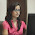## Thursday, October 30, 2014

### Scala high order methods: collect & partition

This post describes the use cases and typical implementation of the Scala collect and partition higher order methods.

Overview
The Scala higher order methods collect, collectFirst and partition are not commonly used, even though these collection methods provide developers with a higher degree of flexibility than any combination of map, find and filter.

TraversableLike.collectFirst
The method create a new collection by applying a partial function to all elements of this traversable collection, such as arrays, list or map on which the function is defined. It signature is
def collect[B](pf: PartialFunction[A, B]): Traversable[B]
The use case is to validate K set (or samples) of data from a dataset. Once validated, these K sets are used in K-fold validation of a model generated through training of an machine learning algorithm: K-1 sets are used for training and the last set is used for validation. The validation consists of extracting K samples arrays from a generic array then test that each of these samples are not too noisy (standard deviation does not exceed a high threshold.
. The first step is to create the two generic functions of the validation: breaking the dataset into K sets, then compute the standard deviation of each set. This feat is accomplished by the ValidateSample trait

  1 2 3 4 5 6 7 8 9 10 11 12 13 14 15 16 17 18 19 val sqr = (x : Double) => x*x trait ValidateSample { type DVector = Array[Double] // Split a vector into sub vectors def split(xt: DVector, nSegments: Int): Iterator[DVector] = xt.grouped(((xt.size/nSegments).ceil).toInt) lazy val stdDev = (xt: DVector) => { val mu = xt.sum/xt.size val var =(xt.map(_ - mu) .map(sqr(_)) .reduce( _ + _))/(xt.size-1) Math.sqrt(var) } def isValid(x: DVector, nSegments: Int): Boolean } 

The first method, split breaks down the initial array x into an indexed sequence of segments or sub-arrays. The standard deviation stdDev is computed by folding the sum of values and sum of squared values. The value is defined as lazy so it is computed on demand once for all. The first validation class ValidateSampleMap uses a sequence of map and find to test that all the data segments extracted from the dataset have a standard deviation less than 0.8

class ValidateWithMap extends ValidateSample {
override def isValid(x: DVector, nSegs: Int): Boolean =
split(x, nSegs).map( stdDev(_) ).find( _ > 0.8) == None
}


The second implementation of the validation ValidateSampleCollect uses the higher order function collectFirst to test that all the data segments (validation folds) are not very noisy. collectFirst requires a PartialFunction to be defined with a condition of the standard deviation.

class ValidateWithCollect extends ValidateSample {
override def isValid(x: DVector, nSegs: Int): Boolean =
split(x, nSegs).collectFirst {
case xt: DVector => (stdDev(xt) > 0.8) } == None
}
}


There are two main differences between the first implementation combining map and find and collectFirst implementation
• The second version requires a single higher order function, collectFirst , while the first version uses map and find.
• The second version throws a MatchErr exception as soon as a data segment does not comply
These two implementations can be evaluated using a simple driver application that takes a ValidateSample as argument.

  1 2 3 4 5 6 7 8 9 10 11 12 13 14 15 16 val rValues = Array.fill(NUM_VALUES)(Random.nextDouble) Try ( new ValidateWithMap(0.8).isValid(rValues, 2) ).getOrElse( false) Try ( new ValidateWithCollect(0.8).isValid(rValues, 2) ) match { case Success(seq) => {} case Failure(e) => e match { case ex: MatchError => {} case _ => {} } } 

TraversableLike.collect
The method collect behavior similar to collectFirst. As collectFirst is a "partial function" version of "find", then collect is the "partial function" version of "filter".

def filter1(x: DVector, nSegments: Int): Iterator[DVector] =
split(x, nSegments).collect(pf)

def filter2(x: DVector, nSegments: Int): Iterator[DVector] =
split(x, nSegments).filter( stdDev( _ ) > ratio)


TraversableLike.partition
The Higher order method partition is used to partition or segment a mutable indexed sequence of object into a two indexed sequences given a boolean condition (or predicate).
def partition(p: (A) ⇒ Boolean): (Repr, Repr)
The test case consists of segmenting an array of random values, along the mean value 0.5 then compare the size of the two data segments. The data segments, segs should have similar size.

final val NUM_VALUES = 10000
val rValues = Array.fill(NUM_VALUES)(Random.nextDouble)

val segs = rValues.partition( _ >= 0.5)
val ratio = segs._1.size.toDouble/segs._2.size
println(s"Relative size of segments \$ratio")


The test is executed with different size of arrays.:
NUM_VALUES  ratio
50      0.9371
1000      1.0041
10000      1.0002
As expected the difference between the two data segments size converges toward zero as the size of the original data set increases (law of large numbers).

References
Scala By Example - M. Odersky - June 2014

1.a pride for me to be able to discuss on a quality website because I just learned to make an article on
cara menggugurkan kandungan

2.I really enjoyed your blog Thanks for sharing such an informative post.
clipping path
clipping path service
background removal
car editing

3.TreasureBox is operated by a group of young, passionate, and ambitious people that are working diligently towards the same goal - make your every dollar count, as we believe you deserve something better.
Check out the best
furniture
tv stand nz
bike stand nz